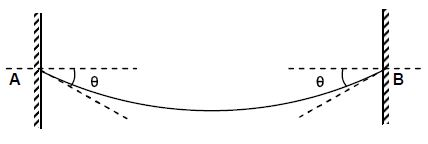# Tension at the lowest point!A heavy string of mass $m$ hangs between two fixed points $A$ and $B$ at the same level. The tangents to the string at $A$ and $B$ are at an angle $\theta$ with the horizontal as shown in the figure. The tension in the string at lowest point is?

×

Problem Loading...

Note Loading...

Set Loading...